# Quadratic Equations: Concepts, Methods and Calculations

in hive-122108 •  5 months ago  (edited)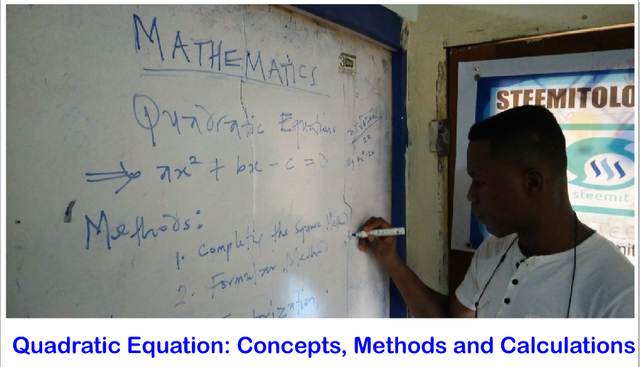In view of the Joint Admission Matriculation Board Examination (JAMB) set up for students aspiring for higher disciplines in the tertiary institutions (universities, polytechnics and colleges) in Nigeria, I decided to organize mathematics tutorial sections for few students whose examinations areas involved mathematics.

When we talk of mathematics, we talk of numbers and variables and it mostly has to do with calculations. Mathematics is really fun when one gets to learn, understand and solve problems. Mathematics have various broad parts of which includes; number theory, geometry, analysis and algebra.

By definition, algebra which is a unifying aspect of nearly all mathematics refers to the process of studying mathematical symbols and variables, together with the principles of manipulating and calculating for these symbols. It involves elementary equations as well as abstractions studying (groups, rings etc).

Furthermore, there are two main types of algebra which are: modern (or abstract) algebra and elementary algebra. Whereas the elementary algebra is the most basic part of algebra,there are mostly considered to be useful for any study of mathematics, economics, engineering or science etc.

On the other hand, modern (or abstract) algebra as the name implies has to do mainly with the study of abstractions such as fields, rings etc. Being an advanced part of mathematics, it is mostly studied by professionals and advanced mathematicians.

A quadratic is a polynomial function with one or more variables with the second degree being the highest-degree term in the equation. Thus, a quadratic function is a quadratic polynomial of degree 2. A quadratic function always contains a constant with at least one of the coefficients of the highest-degree term being a non-zero number for a one-variable case function and being zero for a bivariate case.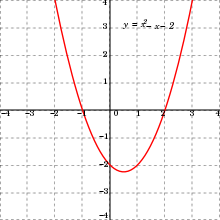A quadratic polynomial with two real roots (crossings of the x axis) and hence no complex roots: WikiMedia

There are univariate and bivariate cases of quadratic functons. Whereas the univariate quadratic function contains just x-terms, the bivariate case consists of both x- and y- terms. It should be noted that the graph of a univariate quadratic function is usually a parabola whose axis of symmetry is parallel to the y-axis.

But whenever the quadratic function is set to be equal to zero, the result is a referred to as a quadratic equation. The solutions to the univariate equation are called the roots of the univariate function.

Furthermore, a quadratic equation usually involves only one unknown and contains only powers of x that are usually positive integers. It can also be said to be second order polynomial equation. The x-term stands for the an unknown which is being calculated for.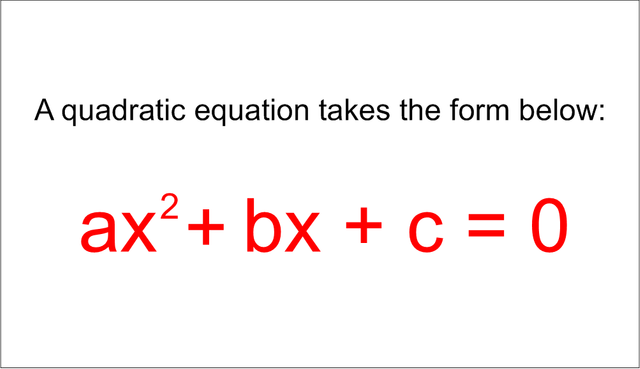While the coefficients a, b, and c are real numbers and it should be noted that 'a-variable' is not equal to zero; reason being that if 'a' is equal to zero, the equation is no more a quadratic rather it becomes a linear equation. More so, to be more specific about the coefficients, 'a' is called the quadratic coefficient, 'b' refers to the linear coefficient while 'c' is termed the constant or free term being that no variable is attached to it.

The values of x that satisfy the equation are called solutions of the equation, and roots or zeros of its left-hand side. A quadratic equation has at most two solutions. If there is no real solution, there are two complex solutions. If there is only one solution, one says that it is a double root. A quadratic equation always has two roots, if complex roots are included and a double root is counted for two. A quadratic equation can be factored into an equivalent equation ref..

The solutions to any quadratic equation that is, the value of x are usually two numbers which could either be negative or positive. These solutions are also known as the roots of the equation.

There are majorly four methods of solving quadratic equations and they include:

Completing the Square Method
This method is often employed in any computation involving quadratic polynomials and it is mostly used in calculating for the solutions of quadratic equations. The method is also useful in the derivation of the quadratic formula as well as graphing quadratic functions.

The first step of applying this method in solving quadratic equations is to divide each side of the equation by "a" which represents the coefficient of the degree 2 term. Second step is to subtract the constant term "c/a" from both sides of the equation. Then add the square of one-half of "b/a", the coefficient of x, to both sides of the equation. This "completes the square", converting the left side into a perfect square. Next is to produce two linear equations by equating the square root of the left side with the positive and negative square roots of the right side and then calculate each of the two linear equations to get two solutions.

Factorization Method
Factorization has to do with the decomposition of a mathematical object into products of smaller or simpler objects. Note worthy is that every function may be factored into the composition of a surjective function with an injective function. This method in mathematics was first applied by the ancient Greek mathematicians in the case of integers and with this, they were able to come up with the fundamental theorem/ law of arithmetic. The theorem states that every positive integer may be factored into a product of prime numbers, which cannot be further factored into integers greater than 1.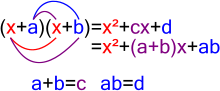WikiMedia

Generally, the factorization method involves writing a number or another mathematical object as a product of other factors, usually smaller or simpler objects of the same kind. Taking an example, 2 × 9 is a factorization of the integer 18 while 6 x 6 is a factorization of the integer 36.

Studies show that in elementary algebra, factoring a polynomial reduces the problem of finding its roots to finding the roots of the factors. More so, polynomials with coefficients in the integers possess the unique factorization property. Factorization can be carried out with root-finding algorithms.

While in secondary school, this method was always referred to as the Almighty Formula and it appeared to be the most simple method for calculating quadratic equations. The quadratic formula is a formula that provides the solution(s) to a quadratic equation. Mathematically, it is given as:Source - WikiMedia

where :
b = Linear coefficient
c = Constant or free term

## Application of Formula Method: Examples

Example One: Find the roots of the equation: 2x2 + 4x - 4 = 0
Solution:
a = 2; b = 4; c = -4
substituting in the formula gives;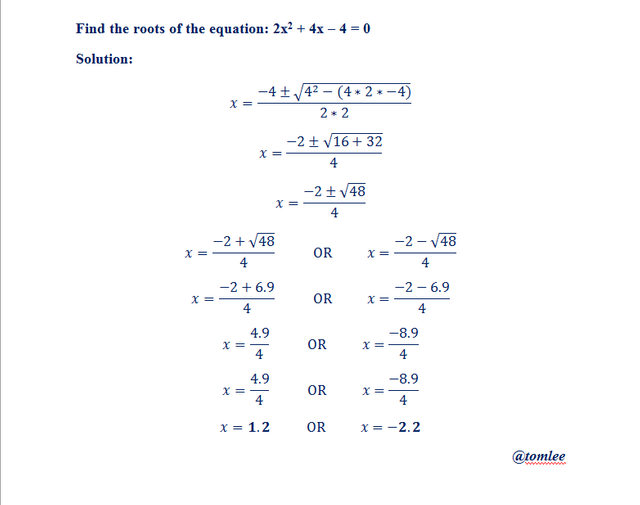Example 2: Find the roots of the equations: x2 + 6x - 16 = 0
Solution:
a = 1; b = -6; c = -16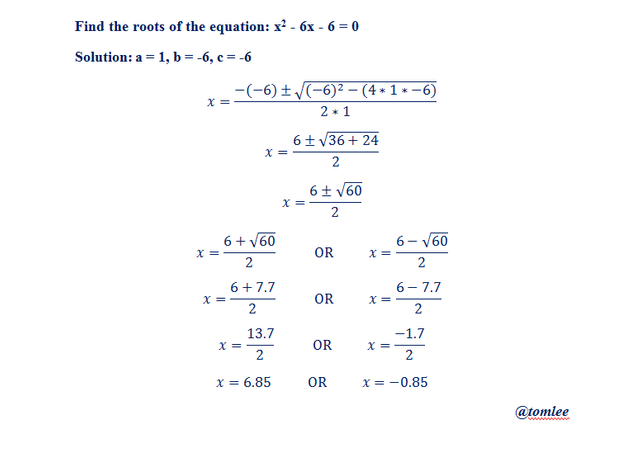I hope you found this interesting!

Sort Order:
·  5 months agoJoin the community in our migration to Hive, a community built blockchain for the community. All Steem account holders will receive equivalent stake on the new Hive blockchain.